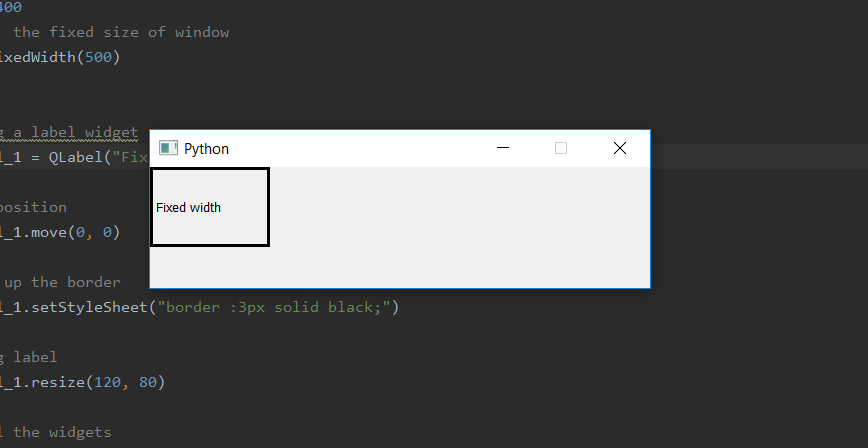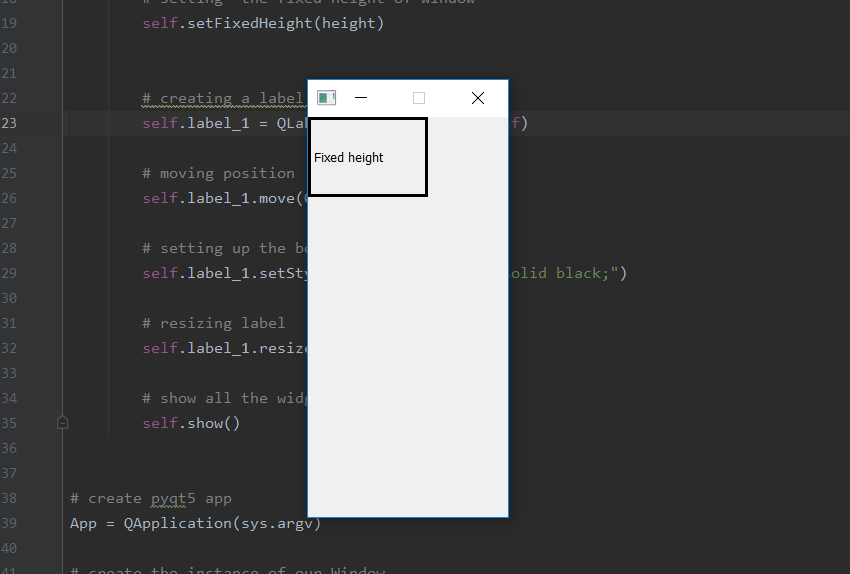Related Articles

# PyQt5 – Set fix window size for height or width

• Last Updated : 26 Mar, 2020

When we create a window, by default the window size is resizable although, we can use `setFixedSize()` method to set the fixed size of the window but if we want to set only fix length of height or width only we cant use this method. We want to set one length fixed and other be variable in order to do so we have to use `setFixedWidth()` method to set fix length of width and `setFizedHeight()` method to set fix length of height.

Syntax :

```self.setFixedWidth(width)
self.setFixedHeight(height)
```

Argument : Both take integer as argument.

Action performed:
`setFixedWidth()` sets the constant width.
`setFixedWidth()` sets the constant height.

Code for fixed width –

 `# importing the required libraries`` ` `from` `PyQt5.QtCore ``import` `*` `from` `PyQt5.QtGui ``import` `*` `from` `PyQt5.QtWidgets ``import` `*` `import` `sys`` ` ` ` `class` `Window(QMainWindow):``    ``def` `__init__(``self``):``        ``super``().__init__()`` ` ` ` `        ``# set the title``        ``self``.setWindowTitle(``"Python"``)`` ` `        ``width ``=` `500``         ` `        ``# setting  the fixed width of window``        ``self``.setFixedWidth(width)`` ` ` ` `        ``# creating a label widget``        ``self``.label_1 ``=` `QLabel(``"Fixed width"``, ``self``)`` ` `        ``# moving position``        ``self``.label_1.move(``0``, ``0``)`` ` `        ``# setting up the border``        ``self``.label_1.setStyleSheet(``"border :3px solid black;"``)`` ` `        ``# resizing label``        ``self``.label_1.resize(``120``, ``80``)`` ` `        ``# show all the widgets``        ``self``.show()`` ` ` ` `# create pyqt5 app``App ``=` `QApplication(sys.argv)`` ` `# create the instance of our Window``window ``=` `Window()`` ` `# start the app``sys.exit(App.``exec``())`

Output :Code for fixed height –

 `# importing the required libraries`` ` `from` `PyQt5.QtCore ``import` `*` `from` `PyQt5.QtGui ``import` `*` `from` `PyQt5.QtWidgets ``import` `*` `import` `sys`` ` ` ` `class` `Window(QMainWindow):``    ``def` `__init__(``self``):``        ``super``().__init__()`` ` ` ` `        ``# set the title``        ``self``.setWindowTitle(``"Python"``)`` ` `        ``height ``=` `400``        ``# setting  the fixed height of window``        ``self``.setFixedHeight(height)`` ` ` ` `        ``# creating a label widget``        ``self``.label_1 ``=` `QLabel(``"Fixed height"``, ``self``)`` ` `        ``# moving position``        ``self``.label_1.move(``0``, ``0``)`` ` `        ``# setting up the border``        ``self``.label_1.setStyleSheet(``"border :3px solid black;"``)`` ` `        ``# resizing label``        ``self``.label_1.resize(``120``, ``80``)`` ` `        ``# show all the widgets``        ``self``.show()`` ` ` ` `# create pyqt5 app``App ``=` `QApplication(sys.argv)`` ` `# create the instance of our Window``window ``=` `Window()`` ` `# start the app``sys.exit(App.``exec``())`

Output :Attention geek! Strengthen your foundations with the Python Programming Foundation Course and learn the basics.

To begin with, your interview preparations Enhance your Data Structures concepts with the Python DS Course. And to begin with your Machine Learning Journey, join the Machine Learning – Basic Level Course

My Personal Notes arrow_drop_up